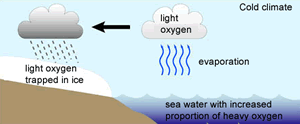Vostok ice cores.Vostok ice core samples reveal temperature patterns over the last 400,000 years. How can we determine the temperature thousands of years ago from air and ice samples deep in the Antarctic snow? The answer is isotopes. Oxygen has three naturally occurring isotopes: 16O, 17O, and 18O, where the 16, 17 and 18 refer to the atomic mass made up of 8 protons and 8 neutrons, 8 protons and 9 neutrons and 8 protons and 10 neutrons respectively. The most abundant of these isotopes is 16O, while a small percentage exist as 18O and an even smaller percentage as 17O. Analysing the ratio between 18O and 16O can provide a way of determining paleotemperature. Analysis is quite complex so we will try and simplify the explanation as much as possible. The 18O isotope is two neutrons heavier than the16O isotope. This causes the water molecule, containing the 18O isotope, to be heavier than the water molecule containing the 16O isotope, hence more energy is required to vaporise the heavier water (H218O) . Because the lighter water molecule (H216O) requires less energy to vaporise the first water vapour formed during evaporation of liquid water has a higher ratio of lighter molecules the liquid left behind has a higher proportion of H218O. When water vapour condenses into liquid, the heavier molecule enters the liquid phase in preference to the lighter molecule, while H216O is concentrated in the remaining vapour.As warm air is cooled, water vapour condenses into water . The precipitation removes a greater proportion of heavy water molecules (H218O) from the vapour, leaving progressively more H216O-rich water vapour. However the degree to which this happens is temperature dependant and we find that the fraction of 18O/16O, in the precipitate, gets lower as the temperature decreases. It is this ratio that is measured in the ice cores and used to determine temperatures of past decades.Limestone can also be used to derive the possible temperature many millions of years ago. Limestone is formed from the calcium carbonate trapped in the shells of microorganisms. Water and carbon dioxide combine to form carbonate ions according to the equation below H2O + CO2 => H2CO3 Microorganisms then react a calcium ion with the carbonate ion to form solid calcium carbonate, according to the equation below, which they use to form their shell. Ca2+ + CO3-2 => CaCO3 Since the water provides one of the oxygens in the calcium carbonate the isotope ratio in the limestone should be the same as that in the water in which the organisms came from.Interesting patterns in the data include the extreme increases and decreases in temperature preceding and following the interglacial phases (the five high temperature phases in the graph). What do you think caused the temperature spikes shown on the graph above? Looking at the graph you can see that rise in carbon dioxide lags a few hundred years behind temperature increase. Is this an indication that carbondioxide does not cause temperature increase? Explain. Explain how the temperature thousands of years ago can be deduced from the air and ice samples in the core? How can limestone be used to also obtain the temperature of the atmosphere thousands of years ago? Looking at the graph of carbon dioxide and temperature above, some event has caused an increase in temperature prior to an increase in carbon dioxide. Suggest one possible explanation as to why the carbon dioxide levels increased after a temperature rise. The oceans are said to act as heat sinks. What is a heat sink and how would it influence a rise in temperature? Look at the graph above. As the temperature increases, positive feedback mechanisms continue to increase the temperature. The rise in carbon dioxide, after a temperature increase, continues to fuel this increase in by acting as a greenhouse gas. Where does this carbon dioxide come from? What is a positive feedback? Explain ways in which the temperatures can return to normal after such spikes. Think about negative feedback mechanisms. One might be that the increase in temperature results in increased evaporation which causes an increase in cloud cover thus resulting in greater solar energy being reflected back into space.